# Algebra II : Quadratic Functions

## Example Questions

1 2 7 8 9 10 11 12 13 15 Next →

### Example Question #3 : Graphing Hyperbolic Inequalities

Which inequality does this graph represent?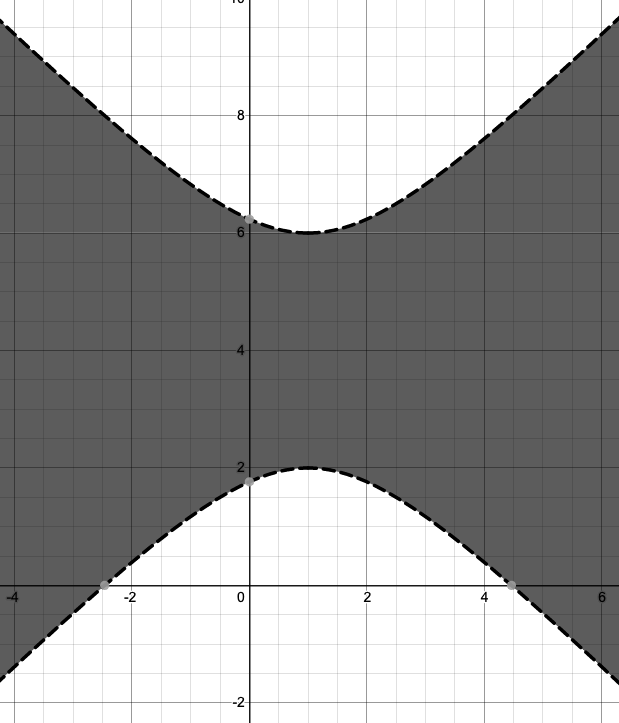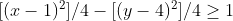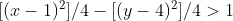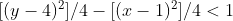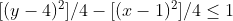Explanation:

The equation for a horizontal hyperbola is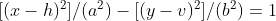. The equation for a vertical hyperbola is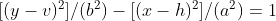. In both,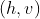is the center of the hyperbola. Hyperbolic inequalities use an inequality sign rather than an equals sign, but otherwise have the same form as hyperbolic equations. The graph shows a vertical hyperbola, so in its corresponding inequality the y-term must appear first. The center is shaded, so the left side of the graph’s corresponding inequality (the side containing the variables x and y) is less than the constant on the right side. The lines are dashed rather than solid, so the inequality sign must be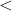rather than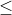.

### Example Question #4 : Graphing Hyperbolic Inequalities

Which of the following inequalities is not hyperbolic?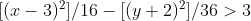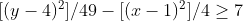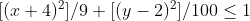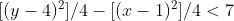Explanation:

The equation for a horizontal hyperbola is. The equation for a vertical hyperbola is. Hyperbolic inequalities use an inequality sign rather than an equals sign, but otherwise have the same form as hyperbolic equations. The fact that the right side of the inequality is not equal to 1 does not change the fact thatandrepresent hyperbolas, since THESE can all be simplified to create an inequality with 1 on the right side (by dividing both sides of the equation by the constant on the right side of the inequality.) Answer choiceis the only option in which the two terms on the left side of the inequality are combined using addition rather than subtraction, creating an ellipse rather than a hyperbola. (The equation for an ellipse is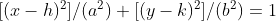.)

### Example Question #5 : Graphing Hyperbolic Inequalities

Which of the following inequalities is not hyperbolic?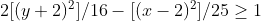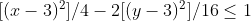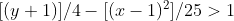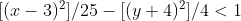Explanation:

The equation for a horizontal hyperbola is. The equation for a vertical hyperbola is. Hyperbolic inequalities use an inequality sign rather than an equals sign, but otherwise have the same form as hyperbolic equations. The presence of coefficients inand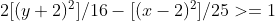does not change the fact thatandrepresent hyperbolas, since both can be simplified to remove those coefficients (by dividing the numerator and denominator of terms with coefficients by those coefficients.) Answer choiceis missing an exponent of 2 on the first term in the inequality, and therefore does not match the form of a hyperbola.

### Example Question #6 : Graphing Hyperbolic Inequalities

Which inequality does this graph represent?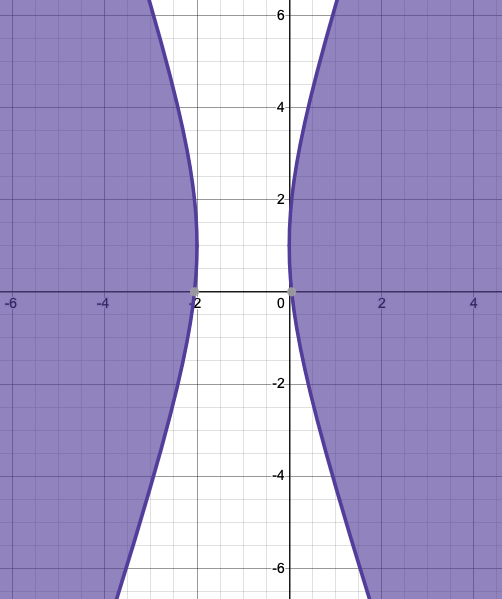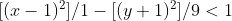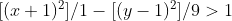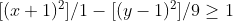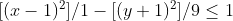Explanation:

The equation for a horizontal hyperbola is. The equation for a vertical hyperbola is. In both, (h, v) is the center of the hyperbola. Hyperbolic inequalities use an inequality sign rather than an equals sign, but otherwise have the same form as hyperbolic equations. The graph shows a horizontal hyperbola, so in its corresponding inequality the x-term must appear first. The center is not shaded, so the left side of the graph’s corresponding inequality (the side containing the variables x and y) is greater than the constant on the right side. The lines are dashed rather than solid, so the inequality sign must be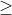rather than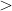. The center lies at (-1, 1), so x must be followed by the constant 1, and y must be followed by the constant -1.

### Example Question #7 : Graphing Hyperbolic Inequalities

Which inequality does this graph represent?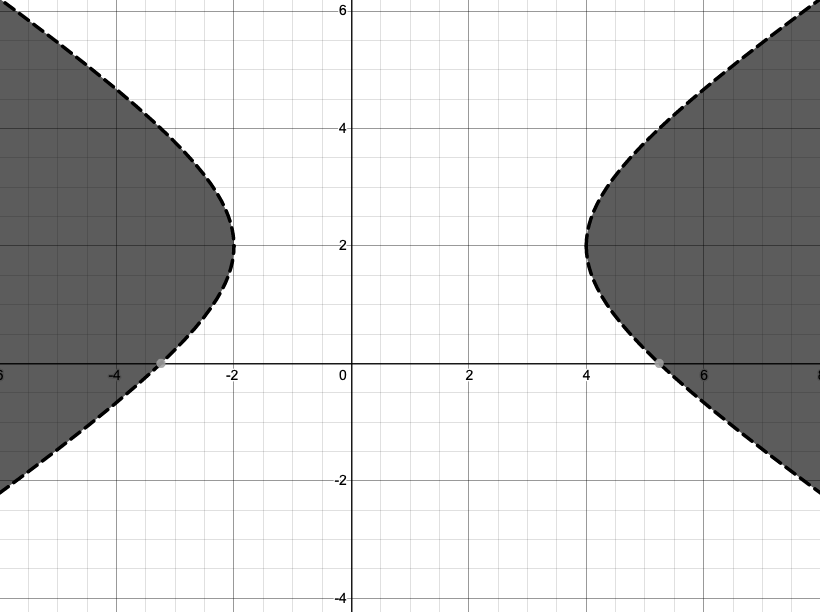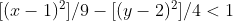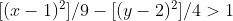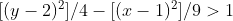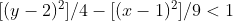Explanation:

The equation for a horizontal hyperbola is. The equation for a vertical hyperbola is. In both, (h, v) is the center of the hyperbola. Hyperbolic inequalities use an inequality sign rather than an equals sign, but otherwise have the same form as hyperbolic equations. The graph shows a horizontal hyperbola, so in its corresponding inequality the x-term must appear first. The center is not shaded, so the left side of the graph’s corresponding inequality (the side containing the variables x and y) is greater than the constant on the right side.

### Example Question #8 : Graphing Hyperbolic Inequalities

Which inequality does this graph represent?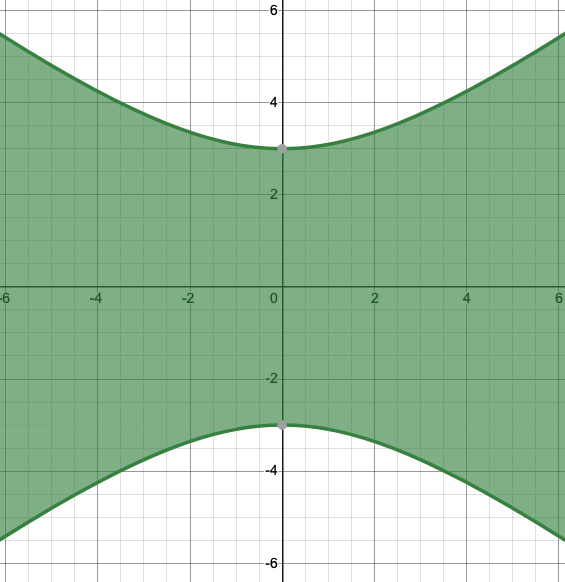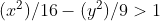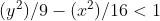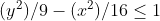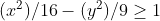Explanation:

The equation for a horizontal hyperbola is. The equation for a vertical hyperbola is. In both, (h, v) is the center of the hyperbola. Hyperbolic inequalities use an inequality sign rather than an equals sign, but otherwise have the same form as hyperbolic equations. The graph shows a vertical hyperbola, so in its corresponding inequality the y-term must appear first. The center is (0, 0), so neither x nor y can be followed by a constant. The center is shaded, so the left side of the graph’s corresponding inequality (the side containing the variables x and y) is less than the constant on the right side. The lines are solid rather than dashed, so the inequality sign must berather than.

### Example Question #9 : Graphing Hyperbolic Inequalities

Which graph represents the inequality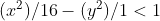?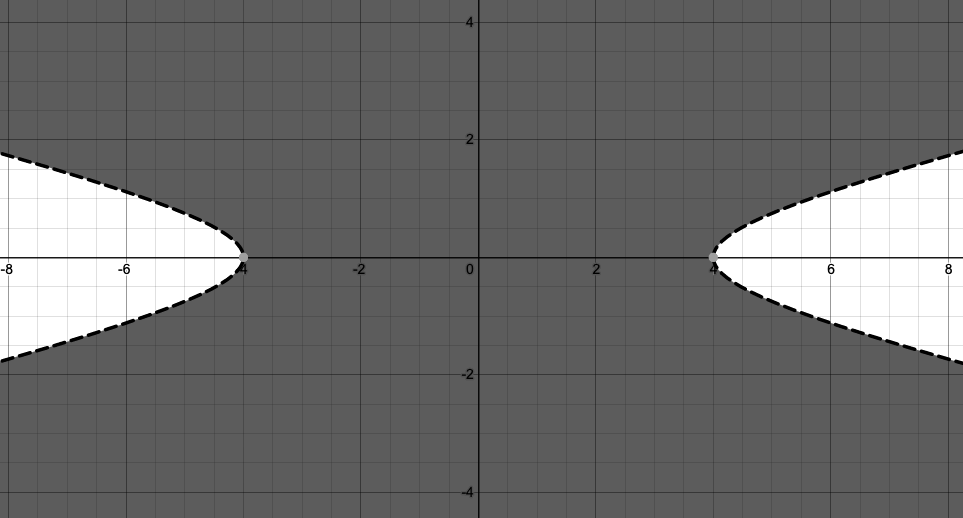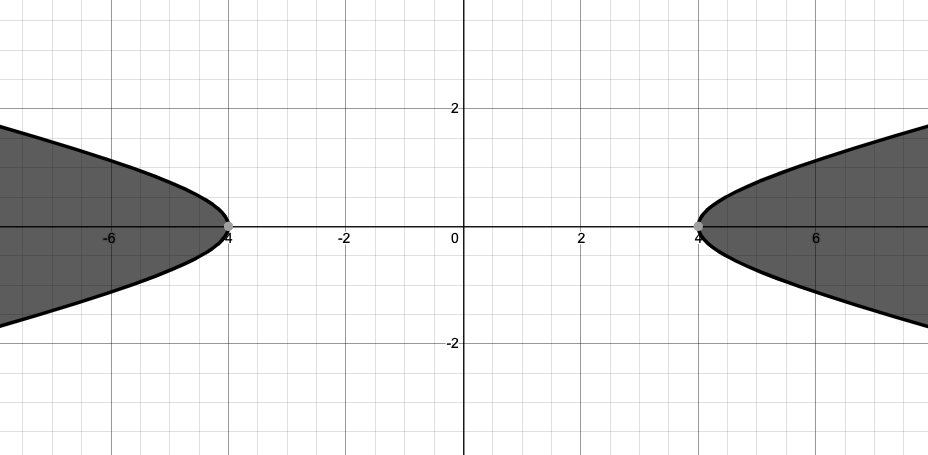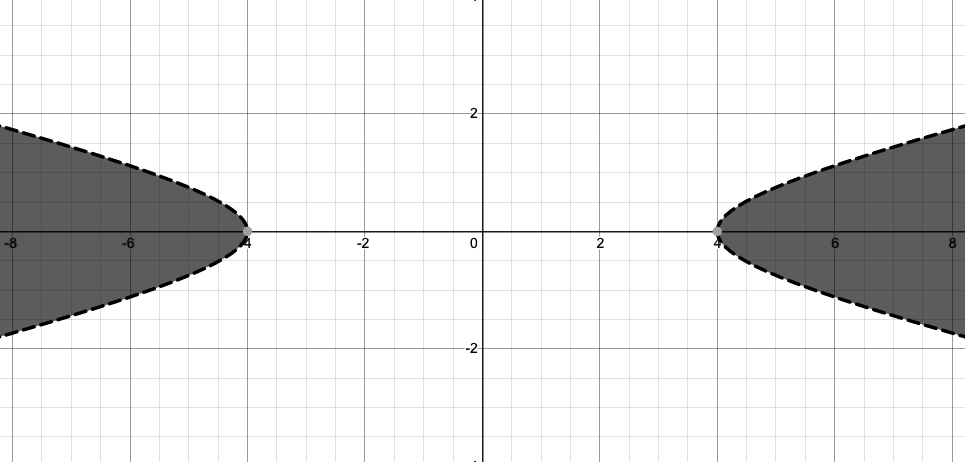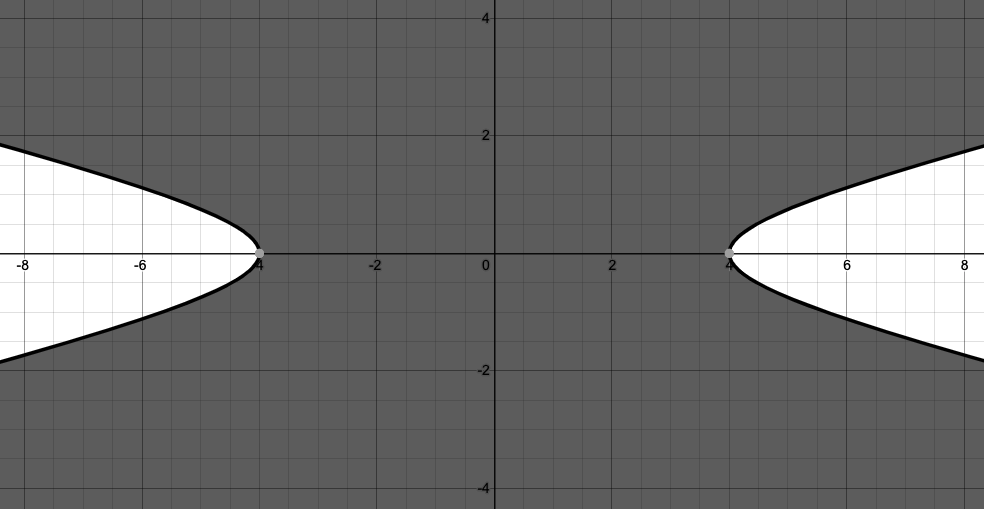Explanation:

The equation for a horizontal hyperbola is. The equation for a vertical hyperbola is. In both, (h, v) is the center of the hyperbola. Hyperbolic inequalities use an inequality sign rather than an equals sign, but otherwise have the same form as hyperbolic equations. Neither the x nor the y in the inequality are followed by constants, so the graph must be centered on (0,0). The x-term appears first, so the graph must show a horizontal hyperbola. The inequality sign israther than, so the lines must be dashed rather than solid. The left side is less than rather than greater than the constant, so the center must be shaded.

### Example Question #10 : Graphing Hyperbolic Inequalities

Which graph represents the inequality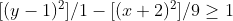?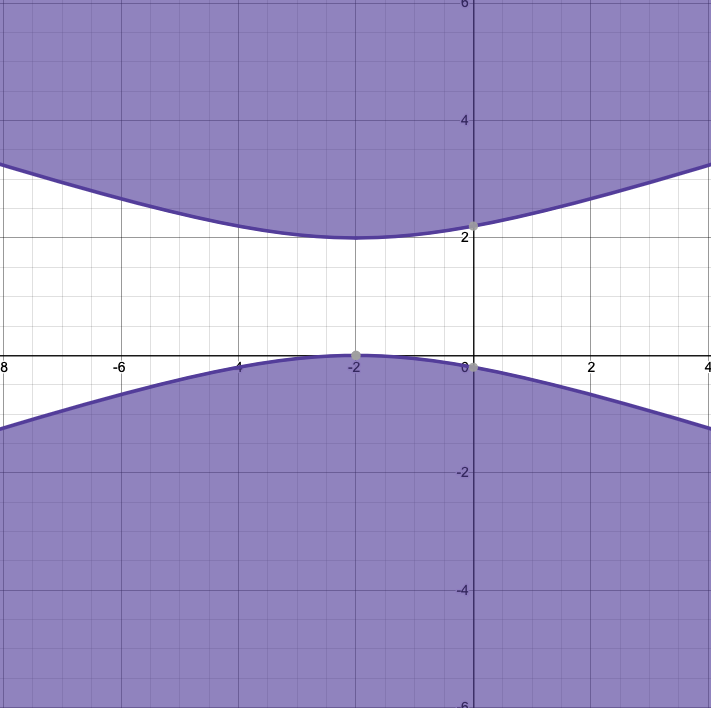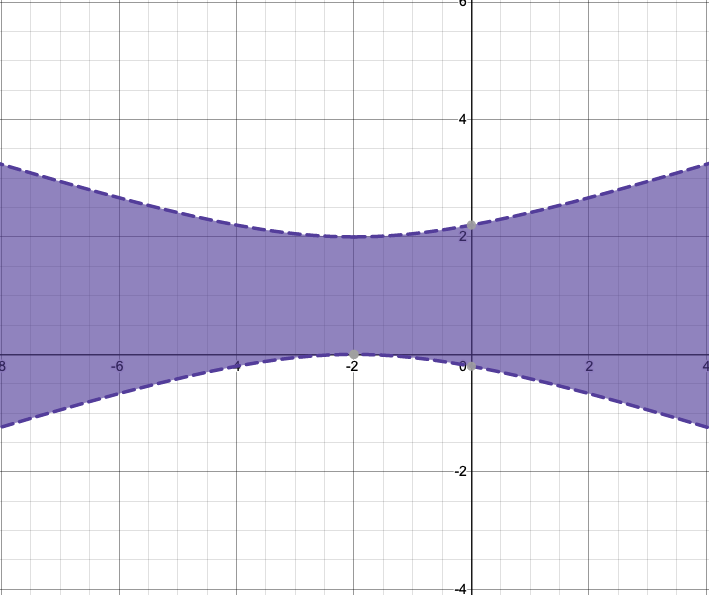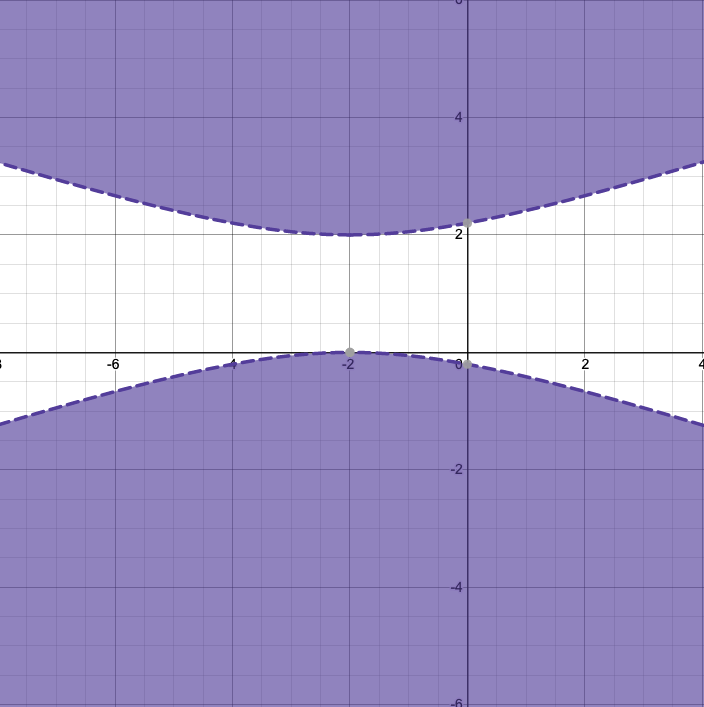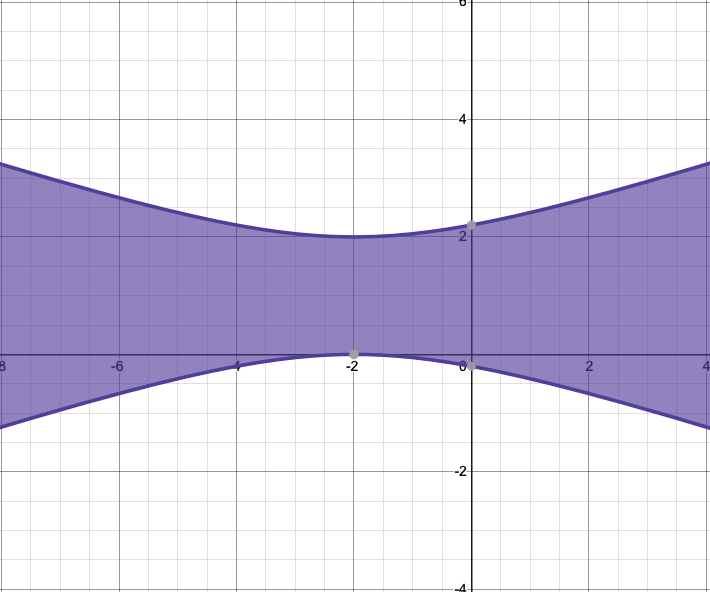The equation for a horizontal hyperbola is. The equation for a vertical hyperbola is. In both, (h, v) is the center of the hyperbola. Hyperbolic inequalities use an inequality sign rather than an equals sign, but otherwise have the same form as hyperbolic equations. The x and y in the inequality are followed by the constants 2 and -1 respectively, so the graph must be centered on (-2, 1). The y-term appears first, so the graph must show a vertical hyperbola. The inequality sign israther than, so the lines must be solid rather than dashed. The left side is greater than rather than less than the constant, so the center must not be shaded.